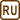## Polarization by Total Internal Reflection

### Task number: 2329

The angle of total internal reflection for transparent enamel is 58°. What is the index of refraction of enamel?

Determine the polarization angle of diamond with index of refraction 2,42.

• #### Hint 1

How does the polarization by reflection work and which relation holds for Brewster’s angle of incidence?

• #### Solution

The light must come under a particular angle of incidence, so called Brewster’s angle αB, to be reflected with complete polarization. At this angle reflected and refracted rays form an angle of 90°. We can get the following relation:

$\alpha_\mathrm{B}+ 90° + \beta = 180°.$

For detailed derivation see the Hint 1 solution.

Simplifying we get:

$\beta = 90° − \alpha_\mathrm{B}.$

Modifying the Snell’s law, we get:

$\frac{\sin\, \alpha_\mathrm{B}}{\sin\, \beta}=\frac{\sin\, \alpha_\mathrm{B}}{\sin\,(90° − \alpha_\mathrm{B})}=\frac{\sin\, \alpha_\mathrm{B}}{\cos\,\alpha_\mathrm{B}}=\mathrm{tg}\,\, \alpha_\mathrm{B}= \frac{n_\mathrm{2}}{n_\mathrm{1}}.$

As the task assignment did not mention differently, we assume that the problem is happening in vacuum or in air, so we can substitute for n1 = 1.

$\mathrm{tg}\,\, \alpha_\mathrm{B}= \frac{n_\mathrm{2}}{1},$

Thus:

$\mathrm{tg}\,\, \alpha_\mathrm{B}= n_\mathrm{2}.$

We can substitute for the Brewster’s angle αB = 58° from the assignment and calculate the index of refraction of enamel:

$n_\mathrm{2}=\mathrm{tg}\,\,58°\dot=\,1{,}6.$

We can also calculate Brewster’s angle for diamond with the index of refraction n2 = 2,42:

$\mathrm{tg}\,\, \alpha_\mathrm{B}= n_\mathrm{2} = 2{,}42,$ $\alpha_\mathrm{B} \dot=\,68°.$